Mobile QR Code1. (Department of Electronic and Electrical Engineering, POSTECH (Pohang University of Science and Technology), Pohang 37673, Korea )
2. (Samsung Electronics, Hwaseong 445-701, Korea )

Tomlinson-Harashima precoding, reflective channel, current comparator, 2 UI feedback loop delay constraint

## I. INTRODUCTION

In the asymmetric high speed interface, such as the DRAM and DRAM controller interface, the DRAM chips are commodity products fabricated by using slow technology while the DRAM controllers are fabricated by using fast technology. In such asymmetric interface, because the equalizer operation is performed by the fast operating chips such as the DRAM controller, the DRAM controller performs the transmitter equalization during the write cycle as well as receiver equalization during the read cycle. For the transmitter equalization, the Tomlinson-Harashima precoding (THP) is known to be effective compared to the feed-forward equalization (FFE) in highly reflective channels with a small number of non-zero channel impulse responses such as multi drop DRAM channels (1-4); the THP uses the same number of taps as the non-zero channel impulse response while the FFE requires an infinite number of taps, theoretically. The THP compensates for the transmission loss and reflection by sending a precoded multi-level data of TX_OUT through a transmission line with the transfer function of H(z); the multi-level data TX_OUT is generated by passing the one-bit input data (TX_IN) through a precoder with the forward gain of 1.0 and the loop gain of 1-H(z) (Fig. 1). However, the THP takes a large chip area to implement the digital multipliers and adders (2). The area of the digital multiplier increases significantly as the resolutions of the data and tap coefficient increase.

As an alternative of digital-based THP, an analog implementation of the THP would reduce the chip area significantly by performing analog multiplications and additions. In analog implementation, regardless of the resolution, the multiplication can be performed by only few transistors. However, the analog THP cannot implement the tapped delay line which delays TX_OUT in integer multiples of data periods (UIs). The tapped delay line is required to implement the loop gain (1-H(z)) for the THP operation. The tapped delay line can be easily implemented with digital circuits such as flip-flops.

In this work, an analog digital mixed mode THP is proposed by combining analog circuits, an analog to digital converter (ADC), a digital tapped delay line, and digital to analog converters (DACs).

Section II describes the proposed analog digital mixed mode THP equalizer. Section III explains the circuit implementation. Section IV presents the measured results. Section V concludes this work.

## II. ANALOG-DIGITAL MIXED-MODE THP EQUALIZER

To increase the data rate of the THP, the proposed THP compensates for the 1$^{\mathrm{st}}$ tap with a feed-forward compensation and the remaining taps with a feedback compensation (1) (Fig. 2(a)); this scheme relaxes the constraint on the feedback loop delay up to 2 data periods (UIs) because the loop gain L(z) starts from z$^{-2}$ as represented by Eq. (1). The transfer function of TX_OUT to TX_IN in Fig. 2(a) is 1/H(z) as in Fig. 1. A data period (UI) is around 185 ps at the data rate of 5.4 Gb/s.

##### (1)
L(z) = (h$_{2 }$-h$_{1}$$^{2}$)·z$^{-2}$+ (h$_{\mathrm{3~}}$-h$_{1}$·h$_{2}$)·z$^{-3}$+··· + (h$_{\mathrm{9~}}$-h$_{1}$·h$_{8}$)·z$^{-9}$+ (h$_{\mathrm{10~}}$-h$_{1}$·h$_{9}$)·z$^{-10}$

Fig. 2. (a) A modified THP with the loop delay relaxed to 2UIs, (b) Analog-digital mixed-mode half-rate THP proposed in this work, (c) Analog Tomlinson-Harashima (ATH) engine.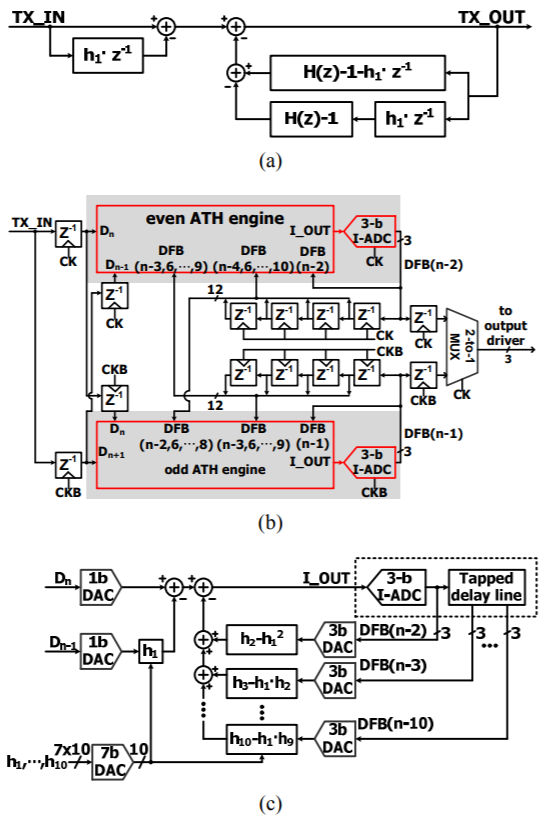This work accepts a full rate data (TX_IN), performs the THP operation by using two half-rate processors, and combines the two processed results with a MUX to generate the full rate TX output (Fig. 2(b)). The half rate processor consists of an analog Tomlinson Harashima (ATH) engine, a 3-bit flash ADC, and a digital tapped delay line. The area-intensive digital multipliers and adders of conventional works ((1), (3)) are replaced by the shaded analog circuits (ATH engine, ADC) to reduce chip area. A half rate clock (CK) is used for the proposed mixed mode half rate THP. The ATH engine accepts the current data input (D$_{\mathrm{n}}$), the preceding data input (D$_{\mathrm{n-1}}$), the decision data (DFB(n-2), DFB(n-3), ···, DFB(n-10)), and generates an analog output (I_OUT). The ADC samples I_OUT at the rising edge of CK to generate a 3-bit decision data (DFB(n-2)), which is applied back to the ATH engine and the tapped delay line. The 3-bit decision data is also sent to a 2-to-1 MUX through a retiming flip-flop. The 3-bit single-ended output of the 2-to-1 MUX is converted to a 3-bit differential data to drive a CML output driver. The CML output driver has 3 PMOS input differential pairs with 3 binary-weighted tail current sources to perform the 8-level THP equalization. One output node of the CML driver is connected to the transmission line for single-ended signaling; a 50 ohm resistor is connected between the output node and ground for TX termination.

The maximum data rate is determined by the loop delay around the ADC and the ATH engine through DFB(n-2). The ADC generates RZ data to reduce the delay time; the RZ data is converted to NRZ data after going through a retiming flip-flop.

The ATH engine converts the digital inputs to analog signals using DACs, performs the filter operations with analog signals and generates the analog output I_OUT using an analog adder (Fig. 2(c)). The ATH engine (Fig. 2(c)) performs the same operation as the modified THP (Fig. 2(a)) except that the ATH engine performs the equalization operation on a quantized analog signal with 3-bit resolution.

## III. CIRCUIT DESCRIPTION

### 1. ATH Engine

The ATH engine shown in Fig. 2(c) has been implemented by connecting eleven NMOS input differential pairs in parallel (Fig. 3); the DP2 performs the feed-forward operation for the 1$^{\mathrm{st}}$ tap h$_{1}$, and DP3~DP11 perform the feedback compensation with DFB(n-2), DFB(n-3), ..., DFB(n-10) as input. The tail currents of the eleven differential pairs are proportional to 1, $\left|\mathrm{h}_{1}\right|,\left|\mathrm{h}_{2}-\mathrm{h}_{1}^{2}\right|,\left|\mathrm{h}_{3}-\mathrm{h}_{2} \cdot \mathrm{h}_{1}\right|,\left|\mathrm{h}_{4}-\mathrm{h}_{3} \cdot \mathrm{h}_{1}\right|,\left|\mathrm{h}_{5}-\mathrm{h}_{4} \cdot \mathrm{h}_{1}\right|,\left|\mathrm{h}_{7}-\mathrm{h}_{6} \cdot \mathrm{h}_{1}\right|$, ···, respectively. The 1$^{\mathrm{st}}$ differential pair (DP1) adds its tail current (I0) to I_OUT when D$_{\mathrm{n}}$=‘1’. When D$_{\mathrm{n-1}}$=‘1’, the 2$^{\mathrm{nd}}$ differential pair (DP2) adds its tail current ($\mathrm{I}_{0} \cdot\left|\mathrm{h}_{1}\right|$) to I_OUT if h1 < 0 and subtracts its tail current ($\mathrm{I}_{0} \cdot\left|\mathrm{h}_{1}\right|$) from I_OUT if h1 > 0. Each of the remaining nine differential pairs (DP3, ···, DP11) is a 3-b binary weighted DAC; the DAC consists of a parallel connection of three NMOS differential pairs with 3-b decision data input (DFB(n-m) for m = 2, 3, ···, 10. In this way, the analog circuit of Fig. 3 performs the same equalization as in Fig. 2(c) and Eq.(1). The sum of the tail currents of the eleven differential pairs is set to 200 $\mu$A. A bleeding current of 400 $\mu$A is added to I_OUT to reduce the voltage change of A_OUT node with the change of digital inputs (D$_{\mathrm{n}}$, D$_{\mathrm{n-1}}$, DFB(n-2), ···, DFB(n-10)); the bleeding current helps increase the maximum data rate because the A_OUT node is loaded by large capacitance. Thus, I_OUT ranges from 400 $\mu$A to 600 $\mu$A with the change of the digital inputs (D$_{\mathrm{n}}$, D$_{\mathrm{n-1}}$, DFB(n-2), ···, DFB(n-10)).

The current output (I_OUT ) of the ATH engine is converted into a digital code by an ADC; this is done because the THP algorithm requires a delay line for the output signal (I_OUT ) and the digital tapped delay line is preferred over the analog delay line due to the noise insensitivity. The ADC is implemented by using a 3-b current mode flash ADC with seven current comparators (Fig. 4). A one tenth of I_OUT is copied to each of the seven current comparators. Each comparator compares the one tenth of I_OUT to the reference current, which ranges from 42.5 $\mu$A to 57.5 $\mu$A in linear steps (IR0 = 42.5 $\mu$A, Δ= 2.5 $\mu$A). The seven comparators generate 7-b thermometer code T<1:7>, which is converted into a 3-b binary code DFB(n-2) by passing through a bubble correction circuit and a binary encoder.

Each current comparator is implemented by cascading a dynamic current integrator and a NOR SR latch (Fig. 5(a)). The basic part of the dynamic current integrator consists of two parallel branches; one branch consists of one current source (MP1) of I_OUT /10, a common gate transistor (MP3) and an integrating capacitor (CP), and the other branch consists of a reference current source (MP2) of IR0+m${\cdot}$Δ, a common gate transistor (MP6) and an integrating capacitor (CN). The current sources (MP1 and MP2) charges the CP and CN with the slew rates of I_OUT (10${\cdot}$CP) and (IR0+m${\cdot}$Δ)CN, respectively, in the evaluation period where the capacitors are discharged to 0 in the pre-charge period. Because the node voltages of DP and DN cannot reach VDD, two CMOS inverters amplify the DP and DN node voltages into rail-to-rail CMOS output signals. The outputs of the CMOS inverters are connected to the 2-input NOR SR latch to become return-to-zero decision data.

The above mentioned basic dynamic current integrator generates a relatively large kickback noise because, as the DP node voltage increases with time during the evaluation period, the current through the current source (MP1) decreases and the drain node voltage of MP1 increases and hence a large kickback noise is induced on the A_OUT node. The kickback noise increases the settling time of the A_OUT node voltage. To reduce the kickback noise, the common gate transistor (MP3) is changed to a differential pair (MP3, MP4) in this work to maintain a constant current through the current source (MP1) and hence a constant voltage at the drain node of MP1; this change reduces the kickback noise of the A_OUT node voltage. Similarly, a differential pair (MP5, MP6) is used for the reference current. At the start of the evaluation period (CKB = ‘1’), all of the MP1 current flows through MP3 and all of the MP2 current flows through MP6 (blue dashed arrow) for the fast charge of the DP and DN node voltages. At the end of the evaluation period where the DP and DN node voltages are much larger than the logic threshold voltage of the CMOS inverter, most of the MP1 and MP2 currents flow through MP4 and MP5, respectively (red solid arrow); this enables to maintain the currents through MP1 and MP2 almost constant and hence the voltages at the drain nodes of MP1 and MP2 almost constant. The kickback noise voltage of the A_OUT node, the current variation of MP1 and the voltage variation of the MP1 node are 19 mVpp, 398 mVpp, 50 $\mu$App in the basic dynamic integrator circuit according to circuit simulation with the MP1 current of 57.5 $\mu$A and the MP2 current of 57.4 $\mu$A; they are 4.6 mVpp, 98 mVpp, 10 $\mu$App in the proposed current comparator with two differential pairs (MP3 and MP4, MP5 and MP6) and a feedback loop. The comparator sensitivity is 6.4 nA in this work according to simulation.

Fig. 5. (a) Circuit diagram of (m+1)th latch-type current comparator, (b) Simulation results of the current comparator circuit.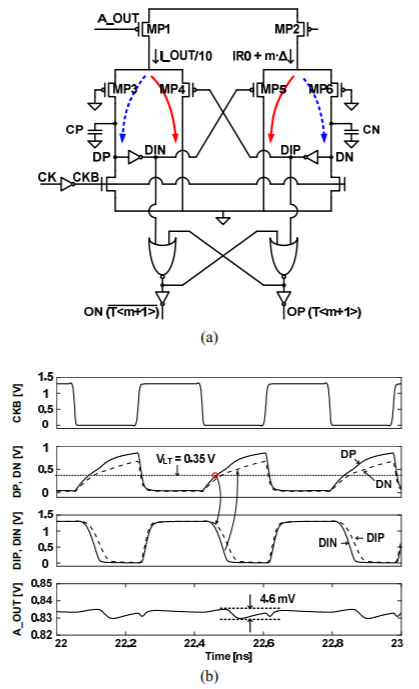Fig. 6. (a) Critical feedback path of the loop around ATH engine and ADC through DFB(n-2) in Fig. 2(b), (b) Simulated delay times.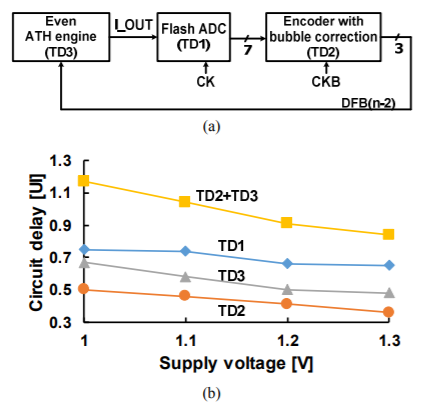To find the critical circuit block which determines the maximum data rate, the loop around the ADC and the ATH engine through DFB(n-2) is re-drawn in Fig. 6(a); the 3b I-ADC of Fig. 2(b) is divided into a flash ADC and an encoder with bubble correction logic. The flash ADC samples I_OUT at the rising edge of CK and generates a RZ data output which is valid while CK=‘1’; the delay time of the RZ data from the rising edge of CK is TD1. The encoder samples the RZ data output of the flash ADC at the falling edge of CK and generates another RZ data output after TD2. The delay time of the ATH engine is TD3. Because half-rate clocks are used for CK and CKB, TD1 must be less than 1 UI and also TD2+TD3 must be less than 1 UI. All the delay times (TD1, TD2 and TD3) are reduced as the supply voltage is increased, as shown in the simulation result of Fig. 6(b). At the supply voltage of 1.3 V, TD1, TD2 and TD3 are 0.65 UI, 0.36 UI and 0.48 UI, respectively at 5.4 Gb/s; both TD1 and TD2+TD3(=0.84 UI) satisfy the timing constraint.

Fig. 8. (a) Measured maximum data rate and energy efficiency vs. supply voltage, (b) Simulated power breakdown.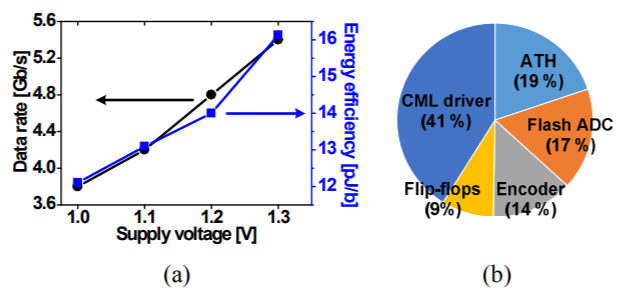## IV. MEASUREMENT RESULTS

The proposed analog digital mixed mode THP equalizer circuit was designed in a 65 nm CMOS process. The active chip area is 0.029 mm$^{2 }$(Fig. 7). The chip consumes 87 mW at the data rate of 5.4 Gb/s with VDD = 1.3 V and 46 mW at the data rate of 3.8 Gb/s with VDD = 1.0 V (Fig. 8(a)).

The proposed THP equalizer chip was tested with a BER tester and a sampling oscilloscope (Fig. 9); the BER tester applies a PRBS-7 full-rate data to the TX_IN pin and a half rate clock to the CK pin of the proposed chip, the TX_OUT pin of the chip is connected to one side of a transmission channel, and the other side of the transmission channel is connected to the input node of the BER tester or an oscilloscope.

A 36-inch FR-4 channel and an 8-inch FR-4 reflective channel with a 4-inch center stub are used for the test (Fig. 10(a), (b)). The unit pulse response (h$_{1}$, h$_{2}$, h$_{3}$, ..., h$_{10}$) of the two channels were measured with the oscilloscope by sending a single pulse from the BER tester with the THP equalizer function turned off in the proposed chip (Fig. 10(c), (d)). As discussed briefly in section I, because the required number of tap is equal to the number of non-zero cursors in the pulse response, two target channels used in this work were covered by 10-tap design. The measured bath-tub curve verifies that the proposed chip works at 5.4 Gb/s in both channels (Fig. 10(e), (f)). It is considered that the maximum data rate is limited by the constraint that the loop delay of the current comparator, the bubble correction logic, the binary encoder and the ATH engine should be less than 2 UIs.

Fig. 10. Measured unit pulse response and bath-tub curve at 5.4 Gb/s (a) 36-inch FR-4 channel, (b) 8-inch FR-4 reflective channel with a 4-inch center stub, (c) Unit pulse response of 36- inch channel, (d) Unit pulse response of 8-inch reflective channel, (e) Bath-tub curve of 36-inch channel, (f) Bath-tub curve of 8-inch reflective channel.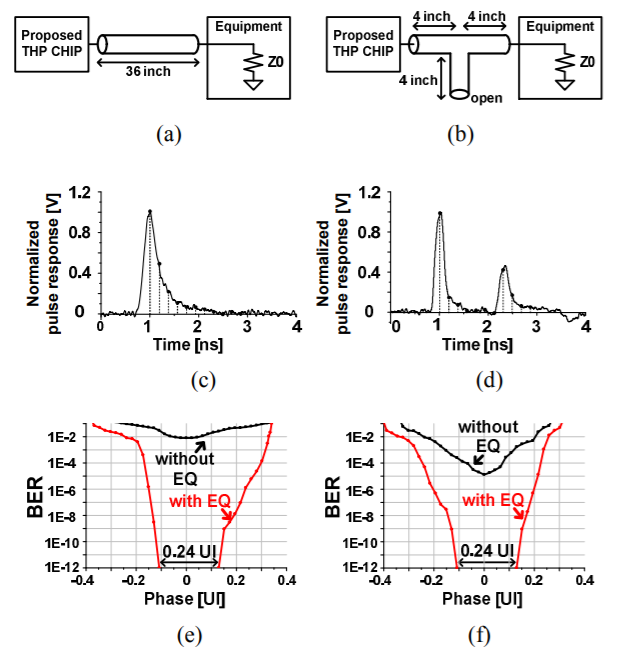With the THP equalizer off, the eyes are closed at the RX input in both channels (Fig. 10(b)). With the THP equalizer on, an 8 level signal can be clearly observed at the TX output (Fig. 11(c)), and open eyes are observed at RX input with the voltage openings of 21.2 mV in the 36-inch FR-4 channels (Fig. 11(d)).

Fig. 11. Measured eye diagrams at 5.4 Gb/s with a 36-inch FR- 4 channel (a) TX_OUT without THP EQ, (b) RX_IN without THP EQ, (c) TX_OUT with THP EQ, (d) RX_IN with THP EQ.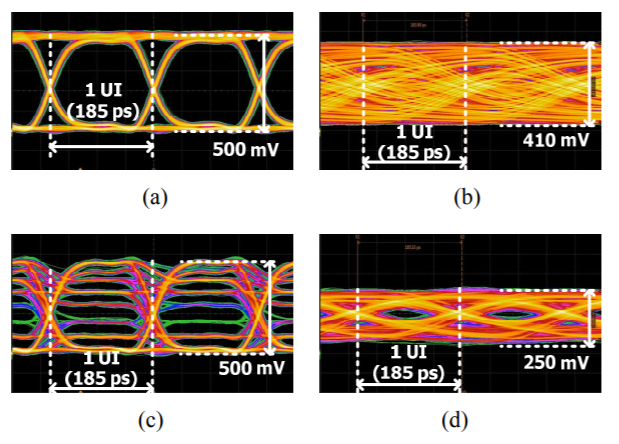The performance comparison shows that the chip area is reduced significantly with the proposed analog-digital mixed-mode THP equalizer compared to the full digital implementation (Table I); the chip areas of (1) and (3) normalized to 65nm process are 9.8 and 5.7 times larger than this work. However, the power is larger in this work than (1) and (3) because of the analog circuits used in this work. The digital multipliers and adders of (1) and (3) are replaced by analog differential pairs and ADCs (Table II); they consume 12.7 mA and 20.7 mA, respectively.

## V. CONCLUSIONS

The Tomlinson-Harashima precoding (THP) equalizer for transmitter was implemented with an analog-digital mixed-mode circuits, because the full digital implementation requires too large chip area to implement a high speed digital multipliers and adders and it is difficult to implement the delay lines required for the THP equalizer with the full analog implementation. To increase the data rate, a half rate THP equalizer was implemented by combining a feed-forward equalizer for the 1$^{\mathrm{st}}$ tap (h$_{1}$) and a THP equalizer for the remaining 9 taps (h$_{2}$, h$_{3}$, ···, h$_{10}$). For the mixed-mode implementation of the half rate THP equalizer, two analog THP engines, two 3-bit ADCs, two 4-tap digital delay lines, a 2-to-1 digital multiplexer and a CML driver were used in this work. The analog THP engine accepts digital inputs, converts the digital inputs into analog signals, performs analog multiplications and additions, and generates an analog output current (I_OUT ); it takes 0.48 data periods (UIs) for the analog THP engine to output the current (I_OUT ) from the digital input. The ADC accepts the current output (I_OUT ) from the analog THP engine and generates a 3-bit binary decision data (DFB(n-2)) by passing I_OUT through a latch type current comparator, a bubble correction logic and a binary encoder; it takes 1.36 UIs for the ADC to generate DFB(n-2) from I_OUT . The latch type current comparator was designed by cascading a dynamic current integrator and a 2-input NOR SR latch; two differential pairs and a feedback loop were used in the dynamic current integrator to reduce the kickback noise. The proposed THP equalizer was implemented in a 65 nm CMOS process. Measured bath-tub curves verify that the fabricated chip works at data rates up to 5.4 Gb/s with a 36-inch FR-4 channel and a 8-inch reflective FR-4 channel with a 4-inch center stub. The chip consumes 87 mW at VDD = 1.3 V. The chip area is 0.029 mm$^{2}$.

### ACKNOWLEDGMENTS

This work was supported in part by the National Research Foundation of Korea (NRF) grant funded by the Korea government (Ministry of Science and ICT) under Grant NRF-2017R1D1A1B03035430, in part by the MSIT (Ministry of Science and ICT), Korea, under the ICT Consilience Creative program (IITP-2019-2011- 1-00783) supervised by the IITP (Institute for Information & communications Technology Planning & Evaluation) and in part by Samsung Electronics.

### REFERENCES

1
Kossel M., Dec 2013, A 10 Gb/s 8-Tap 6b 2-PAM/4-PAM Tomlinson–Harashima Precoding Transmitter for Future Memory-Link Applications in 22-nm SOI CMOS, IEEE Journal of Solid-State Circuits, Vol. 48, No. 12, pp. 3268-32842
Yuminaka Y., Mar 2016, Multiple Valued Signaling for High Speed Serial Links Using Tomlinson Harashima Precoding, in IEEE Journal on Emerging and Selected Topics in Circuits and System, Vol. 6, No. 1, pp. 25-333
Kim T., Nov 2016, A Model Predictive Control Equalization Transmitter for Asymmetric Interfaces in 28nm FDSOI, IEEE Asian Solid State Circuits Conference, pp. 237-2404
Suleiman A., Feb 2014, Model Predictive Control Equalization for High-Speed I/O Links, IEEE Transaction on Circuits and Systems I: Regular Papers, Vol. 61, No. 2, pp. 371-381## Author

Min-Kyun Chae received the B.S. and M.S. degrees in electronic and electrical engineering from the Pohang University of Science and Technology (POSTECH), Pohang, South Korea, in 2012 and 2014, respectively, where he is currently pursuing the Ph.D. degree in electronic and electrical engineering.

His current research interests include high-speed low-power I/O circuits.

Won-Cheol Lee received the B.S. degree in the Department of Electronic and Electrical Engineering from Pohang University of Science and Technology (POSTECH), Korea, in 2015, where he is currently pursuing the M.S and Ph.D.

His current research interests include DRAM controller and high-speed I/O circuits

Eunsung Seo received the Ph.D. degree in electrical engineering from the Pohang University of Science and Technology (POSTECH), Pohang, South Korea.

He has been a Principal Engineer with Samsung Electronics, Hwaseong, South Korea, where he has worked on the circuit design of mobile DRAMs such as LPDDR4, LPDDR3, LPDDR2, MDDR, UtRAM (pseudo-SRAM) since 2002.

His current research interests include memory architecture for high performance and energy-efficient application.

Young-Soo Sohn received the B.S. degree in electrical engineering from Sogang University, Seoul, South Korea, in 1997, and the M.S. and Ph.D. degrees in electrical engineering from the Pohang University of Science and Technology, Pohang, South Korea, in 1999 and 2003, respectively.

He joined Samsung Electronics, Hwaseong, South Korea in 2003, where he has been involved in developing high bandwidth DRAM, such as XDR, GDDR, high-bandwidth memory (HBM), and LPDDR. He is currently a Vice President, where he is an In Charge of Mobile/Graphic DRAM Design Group.

His current research interests include high-speed CMOS circuit design and power integrity/signal integrity (SI).

Kwang-Il Park received B.S., M.S., and Ph.D. degrees in electrical and electronic engineering from the Korea Advanced Institute of Science and Technology (KAIST), Daejeon, Korea in 1993, 1995, and 1999, respectively.

He joined LG Semicon Corporation Ltd., Seoul, South Korea, in 1999, where he was involved in the Rambus DRAM and phase-locked loop (PLL).

Since 2003, he has been with Samsung Electronics, Hwaseong, South Korea. He is a Senior Vice President with the DRAM Design Division.

His current research interests include high-speed, high-density and low-power DRAM, and interface design.

Byungsub Kim was born in Busan, South Korea in 1978. He received the B.S. degree in electronic and electrical engineering from the Pohang University of Science and Technology (POSTECH), Pohang, South Korea, in 2000, and the M.S. and Ph.D. degrees in electrical engineering and computer science from the Massachusetts Institute of Technology (MIT), Cambridge, MA, USA, in 2004 and 2010, respectively.

From 2010 to 2011, he was an Analog Design Engineer with Intel Corporation, Hillsboro, OR, USA. In 2012, he joined the Department of Electronic and Electrical Engineering as a Faculty Member, POSTECH, where he is currently an Assistant Professor.

Dr. Kim received the MIT EECS Jin-Au Kong Outstanding Doctoral Thesis Honorable Mentions, the 2009 IEEE JOURNAL OF SOLID-STATE CIRCUITS Best Paper Award, and the Analog Device Inc. Outstanding Student Designer Award from MIT in 2009, and was a co-recipient of the Beatrice Winner Award for Editorial Excellence at the 2009 IEEE International Solid-State Circuits Conference.

Jae-Yoon Sim (M’02–SM’13) received the B.S., M.S., and Ph.D. degrees in electronic and electrical engineering from the Pohang University of Science and Technology (POSTECH), Pohang, South Korea, in 1993, 1995, and 1999, respectively.

From 1999 to 2005, he was a Senior Engineer with Samsung Electronics, Hwaseong, South Korea. From 2003 to 2005, he was a Post-Doctoral Researcher with the University of Southern California, Los Angeles, CA, USA. From 2011 to 2012, he was a Visiting Scholar with the University of Michigan, Ann Arbor, MI, USA. In 2005, he joined POSTECH, where he is currently an Associate Professor. His current research interests include high-speed serial/parallel links, phase-locked loops, data converters, and power module for plasma generation.

Prof. Sim has served on the Technical Program Committees of the IEEE International Solid-State Circuits Conference (ISSCC), Symposium on VLSI Circuits, and the Asian Solid-State Circuits Conference.

He received the Author-Recognition Award at ISSCC 2013 and was a co-recipient of the Takuo Sugano Award at ISSCC 2001.

Hong-June Park (M’88–SM’13) received the B.S. degree in electronic engineering from Seoul National University, Seoul, South Korea, in 1979, the M.S. degree from the Korea Advanced Institute of Science and Technology, Daejeon, South Korea, in 1981, and the Ph.D. degree in electrical engineering and computer sciences from the University of California, Berkeley, CA, USA, in 1989.

From 1981 to 1984, he was a CAD engineer with ETRI, Daejeon. From 1989 to 1991, he was a Senior Engineer with the TCAD Department of INTEL, USA. In 1991, he joined the Electronic and Electrical Engineering Department as a Faculty Member, Pohang University of Science and Technology, Pohang, South Korea, where he is currently a Professor.

His current research interests include CMOS analog circuit design such as high-speed interface circuits, ROIC of touch sensors, and analog/digital beamformer circuits for ultrasound medical imaging.

Prof. Park is a member of IEEK. He served as the Editor-in-Chief of the Journal of Semiconductor Technology and Science, an SCIE journal from 2009 to 2012, as the Vice President of IEEK in 2012, and as a Technical Program Committee Member of ISSCC, SOVC, and A-SSCC for several years.